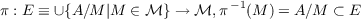#### Vol. 31, No. 3, 1969

 Download this articleFor screen For printingRecent Issues Vol. 325: 1  2 Vol. 324: 1  2 Vol. 323: 1  2 Vol. 322: 1  2 Vol. 321: 1  2 Vol. 320: 1  2 Vol. 319: 1  2 Vol. 318: 1  2Online Archive Volume: Issue:The Journal Subscriptions Editorial Board Officers Contacts Submission Guidelines Submission Form Policies for Authors ISSN: 1945-5844 (e-only) ISSN: 0030-8730 (print) Special Issues Author Index To Appear Other MSP Journals
Representation of L-groups and F-rings

### John Dauns

Vol. 31 (1969), No. 3, 629–654
##### Abstract

Consider an f-algebra A with identity (i.e., a,b,c A, αΛb = 0, c 0 cab = acΛb = 0) over the rationals Q. Let be the maximal l-ideals of A.

THEOREM I. If 1 a A 1∕a A, then each A∕M,M ∈ℳ is a totally ordered division ring, and A can be embedded into a real f-algebra.

THEOREM II. A fiber-bundle or sheaf-like structureis constructed. Assume ∩ℳ = {0}; has the hull-kernel topology. All continuous cross sections σ;ℳ→ E(π σ = identity) form a partial algebra Γ(,E) containing an isomorphic copy of AÂ Γ(,E). Let A = {a A||a| < n1 some integer n}. If

1. 1 a A1∕α A then AÂ Γ(,E), where Â is order dense in Γ(,E). If in addition
2. A is complete with respect to the absolute value |a|,a A,

then AÂ = Γ(,E).

Primary: 06.90
##### Milestones